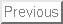How Long is Long?

Today's PCs are typically 32-bit machines, so standard integer data types supports integers roughly in the range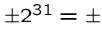2,147,483,648. Thus we can safely count up to a billion or so with standard integers on conventional machines.

We can get an extra bit by using unsigned integers.

Most programming languages support long or even long long integer types, which define 64-bit or occasionally 128-bit integers.9,223,372,036,854,775,808, so we are talking very large numbers!

The magnitude of numbers which can be represented as floats is astonishingly large, particularly with double-precision. This magnitude comes by representing the number in scientific notation, as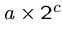. Since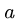and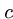are both restricted to a given number of bits, there is still only a limited precision.

Thus don't be fooled into thinking that floats give you the ability to count to very high numbers. Use integers and longs for such purposes.

Representing Enormous-Precision Integers

Representing truly enormous integers requires stringing digits together. Two possible representations are --

• Arrays of Digits - The easiest representation for long integers is as an array of digits, where the initial element of the array represents the least significant digit. Maintaining a counter with the length of the number in digits can aid efficiency by minimizing operations which don't affect the outcome.

• Linked Lists of Digits - Dynamic structures are necessary if we are really going to do arbitrary precision arithmetic, i.e., if there is no upper bound on the length of the numbers. Note, however, that 100,000-digit integers are pretty long yet can be represented using arrays of only 100,000 bytes each.

What dynamic memory really provides is the freedom to use space where you need it. If you wanted to create a large array of high-precision integers, most of which were small, then you would be better off with a list-of-digits representation.

Bignum Data Type

Our bignum data type is represented as follows:


#define MAXDIGITS       100     /* maximum length bignum */

#define PLUS            1       /* positive sign bit */
#define MINUS           -1      /* negative sign bit */

typedef struct {
char digits[MAXDIGITS]; /* represent the number */
int signbit;            /* PLUS or MINUS */
int lastdigit;          /* index of high-order digit */
} bignum;


Adding two integers is done from right to left, with any overflow rippling to the next field as a carry. Allowing negative numbers turns addition into subtraction.


add_bignum(bignum *a, bignum *b, bignum *c)
{
int carry;                      /* carry digit */
int i;                          /* counter */

initialize_bignum(c);

if (a->signbit == b->signbit) c->signbit = a->signbit;
else {
if (a->signbit == MINUS) {
a->signbit = PLUS;
subtract_bignum(b,a,c);
a->signbit = MINUS;
} else {
b->signbit = PLUS;
subtract_bignum(a,b,c);
b->signbit = MINUS;
}
return;
}

c->lastdigit = max(a->lastdigit,b->lastdigit)+1;
carry = 0;

for (i=0; i<=(c->lastdigit); i++) {
c->digits[i] = (char)
(carry+a->digits[i]+b->digits[i]) % 10;
carry = (carry + a->digits[i] + b->digits[i]) / 10;
}

zero_justify(c);
}


Manipulating the signbit is a non-trivial headache. We reduced certain cases to subtraction by negating the numbers and/or permuting the order of the operators, but took care to replace the signs first.

The actual addition is quite simple, and made simpler by initializing all the high-order digits to 0 and treating the final carry over as a special case of digit addition. The zero_justify operation adjusts lastdigit to avoid leading zeros. It is harmless to call after every operation, particularly as it corrects for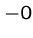:


zero_justify(bignum *n)
{
while ((n->lastdigit > 0) && (n->digits[ n->lastdigit ]==0))
n->lastdigit --;

if ((n->lastdigit == 0) && (n->digits == 0))
n->signbit = PLUS;      /* hack to avoid -0 */
}


Subtraction
Subtraction is trickier than addition because it requires borrowing. To ensure that borrowing terminates, it is best to make sure that the larger-magnitude number is on top.


subtract_bignum(bignum *a, bignum *b, bignum *c) {
int borrow;                     /* anything borrowed? */
int v;                          /* placeholder digit */
int i;                          /* counter */

if ((a->signbit == MINUS) || (b->signbit == MINUS)) {
b->signbit = -1 * b->signbit;
b->signbit = -1 * b->signbit;
return;
}
if (compare_bignum(a,b) == PLUS) {
subtract_bignum(b,a,c);
c->signbit = MINUS;
return;
}
c->lastdigit = max(a->lastdigit,b->lastdigit);
borrow = 0;
for (i=0; i<=(c->lastdigit); i++) {
v = (a->digits[i] - borrow - b->digits[i]);
if (a->digits[i] > 0)
borrow = 0;
if (v < 0) {
v = v + 10;
borrow = 1;
}
c->digits[i] = (char) v % 10;
}
zero_justify(c);
}


Comparison
Deciding which of two numbers is larger requires a comparison operation. Comparison proceeds from highest-order digit to the right, starting with the sign bit:


compare_bignum(bignum *a, bignum *b)
{
int i;                          /* counter */

if ((a->signbit==MINUS) && (b->signbit==PLUS)) return(PLUS);
if ((a->signbit==PLUS) && (b->signbit==MINUS)) return(MINUS);

if (b->lastdigit > a->lastdigit) return (PLUS * a->signbit);
if (a->lastdigit > b->lastdigit) return (MINUS * a->signbit);

for (i = a->lastdigit; i>=0; i--) {
if (a->digits[i] > b->digits[i])
return(MINUS * a->signbit);
if (b->digits[i] > a->digits[i])
return(PLUS * a->signbit);
}

return(0);
}


Multiplication

Multiplication seems like a more advanced operation than addition or subtraction. A people as sophisticated as the Romans had a difficult time multiplying, even though their numbers look impressive on building cornerstones and Super Bowls.

The Roman's problem was that they did not use a radix (or base) number system. Certainly multiplication can be viewed as repeated addition and thus solved in that manner, but it will be hopelessly slow. Squaring 999,999 by repeated addition requires on the order of a million operations, but is easily doable by hand using the row-by-row method we learned in school:


multiply_bignum(bignum *a, bignum *b, bignum *c)
{
bignum row;                     /* represent shifted row */
bignum tmp;                     /* placeholder bignum */
int i,j;                        /* counters */

initialize_bignum(c);

row = *a;

for (i=0; i<=b->lastdigit; i++) {
for (j=1; j<=b->digits[i]; j++) {
*c = tmp;
}
digit_shift(&row,1);
}

c->signbit = a->signbit * b->signbit;
zero_justify(c);
}


Each operation involves shifting the first number one more place to the right and then adding the shifted first number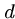times to the total, whereis the appropriate digit of the second number. We might have gotten fancier than using repeated addition, but since the loop cannot spin more than nine times per digit, any possible time savings will be relatively small. Shifting a radix-number one place to the right is equivalent to multiplying it by the base of the radix, or 10 for decimal numbers:


digit_shift(bignum *n, int d)           /* multiply n by 10^d */
{
int i;                          /* counter */

if ((n->lastdigit == 0) && (n->digits == 0)) return;

for (i=n->lastdigit; i>=0; i--)
n->digits[i+d] = n->digits[i];

for (i=0; i<d; i++) n->digits[i] = 0;

n->lastdigit = n->lastdigit + d;
}


Exponentiation is repeated multiplication, and hence subject to the same performance problems as repeated addition on large numbers. The trick is to observe that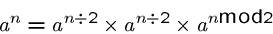so it can be done using only a logarithmic number of multiplications.

Division
Although long division is an operation feared by schoolchildren and computer architects, it too can be handled with a simpler core loop than might be imagined.

Division by repeated subtraction is again far too slow to work with large numbers, but the basic repeated loop of shifting the remainder to the left, including the next digit, and subtracting off instances of the divisor is far easier to program than guessing'' each quotient digit as we were taught in school:


divide_bignum(bignum *a, bignum *b, bignum *c)
{
bignum row;                     /* represent shifted row */
bignum tmp;                     /* placeholder bignum */
int asign, bsign;               /* temporary signs */
int i,j;                        /* counters */

initialize_bignum(c);

c->signbit = a->signbit * b->signbit;

asign = a->signbit;
bsign = b->signbit;

a->signbit = PLUS;
b->signbit = PLUS;

initialize_bignum(&row);
initialize_bignum(&tmp);

c->lastdigit = a->lastdigit;

for (i=a->lastdigit; i>=0; i--) {
digit_shift(&row,1);
row.digits = a->digits[i];
c->digits[i] = 0;
while (compare_bignum(&row,b) != PLUS) {
c->digits[i] ++;
subtract_bignum(&row,b,&tmp);
row = tmp;
}
}

zero_justify(c);

a->signbit = asign;
b->signbit = bsign;
}


This routine performs integer division and throws away the remainder. If you want to compute the remainder of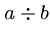, you can always do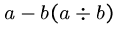.

Numerical Bases and Conversion

The digit representation of a given radix-number is a function of which numerical base is used. Particularly interesting numerical bases include:

• Binary - Base-2 numbers are made up of the digits 0 and 1. They provide the integer representation used within computers, because these digits map naturally to on/off or high/low states.

• Octal - Base-8 numbers are useful as a shorthand to make it easier to read binary numbers, since the bits can be read off from the right in groups of three. Thus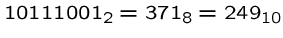. Why do programmers think Christmas is Halloween? Because 31 Oct = 25 Dec!

• Decimal - We use base-10 numbers because we learned to count on our ten fingers.

• Hexadecimal - Base-16 numbers are an even easier shorthand to represent binary numbers, once you get over the fact that the digits representing 10 through 15 are A'' to `'F.''

• Alphanumeric - Occasionally, one sees even higher numerical bases. Base-36 numbers are the highest you can represent using the 10 numerical digits with the 26 letters of the alphabet. Any integer can be represented in base-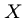provided you can displaydifferent symbols.

Base Conversion

There are two distinct algorithms you can use to convert base-number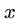to a base-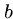number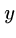--

• Left to Right - Here, we find the most-significant digit offirst. It is the integer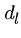such that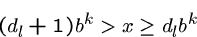where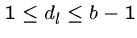. In principle, this can be found by trial and error, although you must to be able to compare the magnitude of numbers in different bases. This is analogous to the long-division algorithm described above.

• Right to Left - Here, we find the least-significant digit offirst. This is the remainder ofdivided by. Remainders are exactly what is computed when doing modular arithmetic. The cute thing is that we can compute the remainder ofon a digit-by-digit basis, making it easy to work with large integers.

Right-to-left translation is similar to how we translated conventional integers to our bignum presentation. Taking the long integer mod 10 (using the % operator) enables us to peel off the low-order digit.

Assigned Problems

110502 (Reverse and Add) - Does repeatedly adding a number to its digit-reversal eventually end on a palindrome?

110503 (The Archeologists' Dilemma) - What is the smallest power of 2 begining with the given digit sequence?

110504 (Ones) - How many digits the smallest multiple of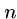such that the resulting digit sequence is all 1s? Why is this possible for every non-multiple of 2 and 5?

110505 (A multiplication game) - What is the right strategy for a two-person digit mulitplication game? Is it recursive/minimax?